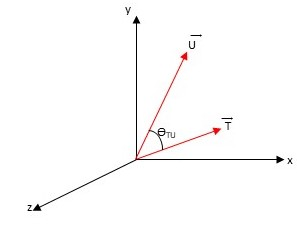# Problem: The figure shows two vectors T_vec and U_vec separated by an angle theta_TU. (Figure 1) You are given that vec{T} = (3,1,0), vec{U} = (2,4,0), and vec{T} × vec{U} = vec{V}. Find the magnitude of V_vec.

###### FREE Expert Solution

Vector Magnitude:

$\overline{)|\stackrel{⇀}{\mathbit{A}}|{=}\sqrt{{{\mathbit{A}}_{\mathbit{x}}}^{2}+{{\mathbit{A}}_{\mathbit{y}}}^{2}+{{\mathbit{A}}_{\mathbit{z}}}^{2}}}$

V = (0,0,10)

99% (421 ratings)###### Problem Details

The figure shows two vectors T_vec and U_vec separated by an angle theta_TU. (Figure 1) You are given that vec{T} = (3,1,0), vec{U} = (2,4,0), and vec{T} × vec{U} = vec{V}.

Find the magnitude of V_vec.